# Experimental Investigation on Transient Pool Boiling Heat Transfer from Rough Surface and Heat Transfer Correlations

Experimental Investigation on Transient Pool Boiling Heat Transfer from Rough Surface and Heat Transfer Correlations
Dr. A. S. College of Agril. Engineering and Technology, MPKV, Rahuri 413722, India

National Institute of Technology Karnataka, Surathkal 575025, India

Corresponding Author Email:
aawalunj@gmail.com
Page:
545-554
|
DOI:
https://doi.org/10.18280/ijht.370223
2 July 2018
|
Accepted:
12 June 2019
|
Published:
30 June 2019
| Citation

OPEN ACCESS

Abstract:

This paper presents the study of transient boiling characteristics on rough copper sample with surface roughness value (Ra) ranging from 0.106 μm to 4.03 μm. The effect of roughness and time constant of exponential heat supply on transient critical heat flux (CHF), maximum heat transfer coefficient (HTC) and onset of nucleate boiling (ONB) is extensively studied. The mechanism of heat transfer in unidirectional scratches of rough sample is discussed. High speed visualization is carried to observe the stages of boiling and bubble dynamics. The HTC correlation developed in this study predicts the present experimental values of HTC with a mean absolute error (MAE) of 9.62 %.

Keywords:

surface roughness, critical heat flux, onset of boiling, high speed visualization

1. Introduction

Enormous amount of heat is generated in the fuel rod due to the exponential escalation of power. The heat generation rate in the fuel rod can be described as $Q \propto e^{\gamma}$ , where γ=t/τ. The time lag between the commencement of the heat generation and onset of boiling is a key factor to keep the surface temperature below the melting point. The physical loss of coolant takes place at a critical heat flux (CHF) due to the transition of the nucleate boiling phase to film boiling. After the onset of boiling, the incidence of transition can vary with the rate of exponential heat generation. Hence, CHF and its corresponding rate of heat reactivity should be monitored to avoid the problem of physical loss of coolant. Since 1957, research is going on to understand the physical mechanism of transient boiling. Attempts have been made to design the experimental facility for different modes of heating, like stepwise, ramp-wise, or exponential heating and for a different type of heating elements like ribbon, wire, cylinder, or plate-type heater. Many investigators have reported the reduction in CHF in variable heat supply, however, few found that CHF is independent of nature of heat supply. The outcomes of the studies carried by the investigators are discussed further. For the first time, Rosenthal  reported that unsteady heat supply does not influence the CHF of pool boiling. Johnson  found that unsteady-state CHF during exponential heating exceeds the CHF at steady-state heat supply. Sakurai and his research group [3-5] examined the boiling characteristics on thin test samples during transient heat supply. Fukuda et al.  remarked that Hydrodynamic Instability (HI) resulted in the reduction of CHF for transient heating condition compared to the steady-state. Transient CHF decreases with decrease in the exponential period upto certain value, after which it increases with further decrease in the exponential period. This phenomenon of the heterogeneous spontaneous nucleation (HSN) is explained by Sakurai et al. . The influence of surface condition of platinum test sample namely, mirror surface (MS) and rough surface (RS), is examined by Fukuda et al.  on pool boiling of water. Deev et al.  investigated the effect of variable heat supply on the mechanism of pool boiling. The variation in the period of boiling regime and CHF with the transient heat supply is also reported. The influence of number of nucleation sites and the thermal inertia of test sample is investigated by Duluc et al. . They found that the steady-state nucleate boiling temperature is lower than that in the transient system of stepwise heat supply. Su et al.  examined the crisis in pool boiling on a plate type heater during exponential heat supply. The moments of onset of nucleate boiling (ONB), Onset of the boiling driven (OBD) and overshoot temperature for different conditions of exponential heat supply are identified. The temperature and heat flux at ONB increased with increase in the period of exponential heat supply. Balakin et al.  analyzed the effect of pressure, test sample size and working fluid on the crisis in the pool boiling during variable heat supply. They reported that test sample size remarkably influences the boiling crisis. Htet et al.  investigated the boiling characteristics on commercial surface (CS), treated surface I (TS-I) and treated surface II (TS-II) under transient condition. In slow transient heat supply, the CHF enhancement for the sample TS-II is reported to be 16 % and 28 % compared to the commercial surface and the sample TS-I, respectively. They also found that the transient condition and system pressure significantly affects the unsteady-state CHF.

It is thus concluded from the literature that pool boiling study under transient condition is investigated mostly with thin, non-lumped test samples. The heat transfer enhancement by choice of passive technique like rough surface under transient heating conditions is rarely examined. The HTC correlation for the surface of wide range of roughness value is also not yet developed for exponential heating condition. The unidirectional scratches are formed on thick copper sample and transient boiling characteristics of water is investigated in the present study. The influence of exponential heating on the ONB as well as CHF is examined. The effect of surface roughness on CHF for transient condition is also reported. The surface roughness effect and the effect of time constant on HTC is examined. A correlation for transient heat transfer coefficient is developed from the experimental independent parameters.

2. Experimentation

2.1 Preparation and characterization of sample

The surface contamination is removed by the sandpaper of 2000 grit. Later, unidirectional scratches are formed on six samples to obtain different surface roughness. During polishing, continuous supply of distilled water is maintained. Thereafter, ethanol, acetone and distilled water are used to clean the sample surface. Surface wettability test by measuring contact angle of water droplet is conducted in goniometer (GBX Digidrop). The droplet of 10 μl is used for its static contact angle measurement. The static contact angle is measured in two ways viz. parallel and perpendicular orientation between camera and unidirectional scratches. The roughness tester (Mitutoyo) is used to measure the surface roughness parameter. Six different locations are selected to measure the roughness values. During the test, perpendicular orientation is maintained between unidirectional scratches and the probe. The evaluation length is 4 mm. The roughness value (Ra) with mean spacing (Sm) of the samples prepare for the trial are given in the Table 1.

Table 1 Roughness parameters in μm

 Ra Sm 0.106 13.2 0.83 26.8 1.87 35.7 3.17 42.2 3.59 44.8 4.03 45.2

## 1.png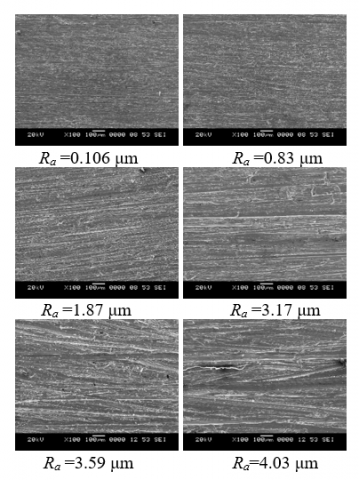Figure 1. SEM images of unidirectional scratches

The SEM images showing the pattern of unidirectional scratches on the surface of the test samples are shown in Figure 1.

2.2 Experimental setup

A boiling chamber with the test section and condenser assembly include in the experimental setup. The schematic of experimental setup and visualization unit is shown in Figure 2. The detachable top and bottom flange are provided to the boiling chamber. The condenser coil is attached to the top flange whereas bottom flange can accommodate the test section assembly. The bulk fluid temperature (Tl) and pressure are measured by the thermocouple and pressure transducer, respectively. The transparent toughened borosilicate glass watch windows of 115 mm diameter and 15 mm thickness are provided to the side wall of boiling chamber to conduct the visualization study by high speed camera. The saturation condition of the distilled water is maintained by the two high density cartridge heaters each of 1000W capacity. The setup is synchronized with high speed camera, temperature module- NI-9213 and NI-9264.

## 2.png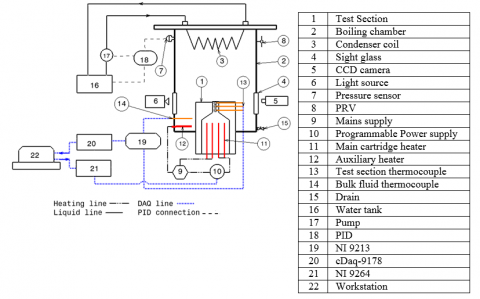Figure 2. Experimental setup

2.3 Test section

Thick copper samples of 20 mm diameter and 20 mm length are prepared and thereafter characterized. The high density cartridge heater of 840W is placed in the heating block. The sample is fixed on the heating block. The screw fitting between sample and heating block ensures the perfect surface contact. The glass wool insulation is provided over the sample and heating block as shown in Figure 3. The high-temperature non-corrosive RTV silicone gasket and O-ring ensures the leak proof assembly. Three K-type sheathed thermocouples of 1 mm diameter are implanted from the top of the sample surface at 2 mm, 6 mm, and 10 mm.

## 3.png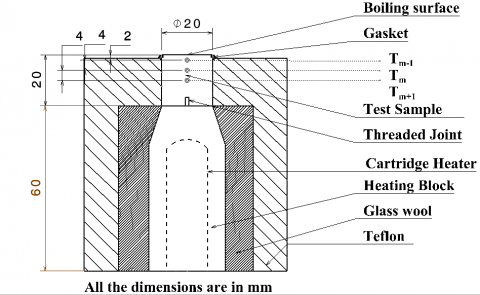Figure 3. Test section assembly

2.4 Experimental procedure

Before each trial, the samples are sequentially cleaned with ethanol, acetone and distilled water. The quantity of the distilled water is maintained constant for all the trials. The gases dissolved in the distilled water are removed by rigorous boiling before the beginning of trial. LABVIEW program controls the analog signal through the hardware of NI-9264. Thus power supply generates the exponentially varying voltage. The experiments are conducted by supplying exponential heat where the parameter called time constant (γ) is maintained at 1. The exponential heat supply (t) is supplied for 10 min. The temperature is recorded at the rate of 5 samples/sec by NI-9213. The pressure control system is adapted for the present experiments to control the system pressure at atmospheric condition.

The assembly of test section is considered as the axisymmetric system. The uniform heat flux from the boiling surface is assumed due to uniform surface characteristics. The sample of temperature readings of Ra=1.87 μm at the different time of acquisition is presented in Figure 4 which shows the temperature distribution obtained by implanted thermocouples. It is observed for all the samples that temperature distribution exhibits a linear fit for each set of readings. The R-squared value is close to unity.

## 4.png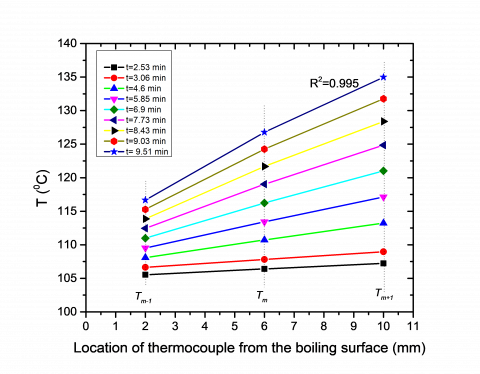Figure 4. Temperature along the length of sample

The heat flux from the boiling surface during exponential heat supply is calculated by Eq. (1).

$q_{t s}^{\prime \prime}=-k_{C u} \frac{T_{m-1}^{t}-T_{m+1}^{t}}{2 \Delta x}$ (1)

where the distance between two thermocouples is given as ∆x.

The temperature of the boiling surface is estimated by using Eq. (2).

$T_{w}^{t}=T_{m-1}^{t}-q_{t s}^{"}\left(\frac{x_{m-1}}{k_{C u}}\right)$ (2)

where, xm-1 is the distance between the boiling surface and the top thermocouple (Tm-1) which is 2 mm, as shown in Figure 3.

Heat transfer coefficient (HTC) between the boiling surface and distilled water is calculated by Eq. (3).

$h_{t s}=\frac{q_{t s}^{\prime \prime}}{\left(T_{w}^{t}-T_{l}\right)}$ (3)

2.5 Uncertainty

The method of propagation of error is used for the uncertainty calculations as discussed in Walunj and Sathyabhama [11, 12]. The uncertainty in temperature measurement is ±0.1 °C whereas the uncertainty in the distance measurement is ±0.0001 m, respectively. For higher temperature range, the uncertainty in the heat flux estimation is 11.91 %. The uncertainty of ±0.55 °C is noticed for the surface temperature estimation. For higher temperature range, 16.20 % uncertainty is found for the estimation of HTC. The uncertainty in the bubble frequency and bubble departure diameter is 10.45 % and 0.05 mm, respectively.

3. Result and Discussion

The parameter called exponential period (τ) was used in the literature to define the exponential heat supply. The value of τ was employed in the range of 2 ms to 20 s where the time of heating (t) was maintained constant. It is clear that selection of τ and t mainly depends on the mass/size of the heating element. It is also obvious that to conduct transient heat transfer study, heating period t must be less than the time required to gain steady-state condition, which varies with the mass of the heating element. It is vague to consider the single time parameter, i.e. τ, in such study without taking the heating period into account. Considering all these facts, present study employs the parameter called time constant γ which is the ratio of heating period and exponential period (γ=t/τ). The rate of exponential heating is identified by the time constant γ and its value is ranging from γ=1 to γ=6. The value of t is 10 min which is constant throughout the study and τ is exponential period. Though various surface roughness parameters viz. Ra, Rq, Sm are measured, Ra is chosen for the nomenclature. The pool boiling characteristics on the smooth and rough surface of a non-lumped sample under exponential heat supply of different period are studied. Experiments are carried in the atmospheric condition of distilled water with a wide range of roughness value. The boiling curves are shown in Figure 5 (a), (b) and (c) for the samples of Ra=0.106 μm, Ra=1.87 μm and Ra=4.03 μm during a different γ, respectively. It is observed that boiling curves shifted towards the right with an increase in γ for all Ra. It indicates that heat transfer increases with decrease in γ. This suggests that heat flux from the boiling sample due to exponential heat supply is inversely proportional to γ. The thermodynamic instability due to a short period of exponential heat supply leads to spontaneous bubble formation. The waiting period between two successive bubbles drops drastically with increase in the heating rate, resulting in the formation of bubble column at all the nucleation sites. Hence, heat transfer from the surface decreases with increase in γ irrespective of its surface roughness.

The effect of surface roughness on the boiling heat transfer at different γ is extensively studied. The boiling curves obtained during γ=2, γ=4 and γ=6 at different Ra are plotted in Figure 6 (a), (b) and (c), respectively. It is observed that heat transfer augments with an increase in Ra for each γ. The nucleation sites have drastically increased due to increase in Ra. The surface wettability improved due to capillary wicking through the unidirectional scratches. The improved liquid supply to the nucleation site has augmented the heat transfer

## 5aa.png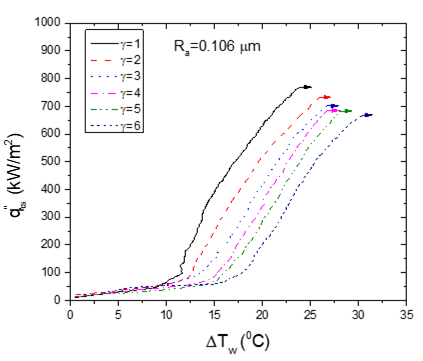(a)

## 5b.png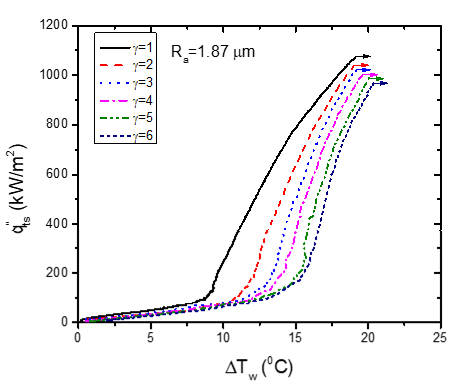(b)

## 5c.png(c)

Figure 5. Boiling curves of the samples (a) Ra=0.106 μm (b) Ra=1.87 μm (c) Ra=4.03 μm at different γ

## 6a.png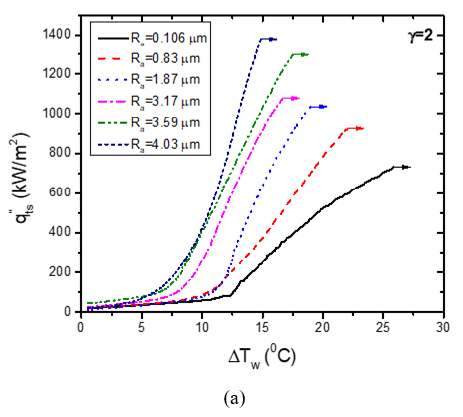## 6b.png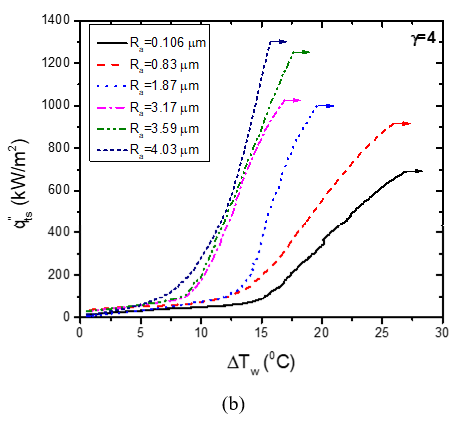## 6c.png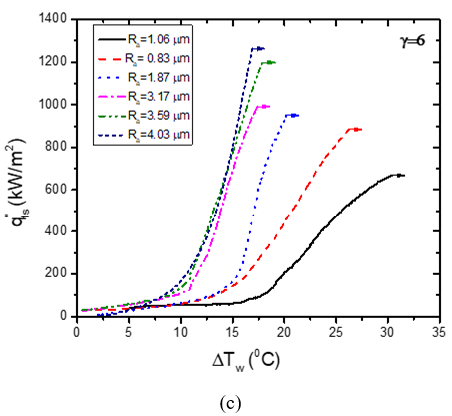Figure 6. Boiling curves obtained during (a) γ=2 (b) γ=4 (c) γ=6 at different Ra

Either homogeneous, heterogeneous nucleation theory or activation of the pre-existing vapor nuclei trapped in cavities can explain ONB. The classical equation can be derived to estimate the rate of formation of critical size vapor nuclei per unit volume within the bulk of a pure liquid. As suggested by Duluc et al. , homogeneous or heterogeneous nucleation theory cannot be considered for the formation of a bubble in the highly wetting fluid. It is believed that activation of pre-existing vapor nuclei is the only cause of bubble formation for the present experimental condition. The study of the compound effect of Ra and γ on ONB temperature is done and values are plotted in Figure 7. It is noticed that ∆TONB is a strong function of Ra and γ where ∆TONB decreases with increase in Ra and it increases with increase in γ. For γ=6, ONB temperature of Ra=0.106 μm, Ra=0.83 μm, Ra=1.87 μm, Ra=3.17 μm, Ra=3.59 μm and Ra=4.03 μm increased by 53.7%, 38.46%, 39.16%, 20.8%, 32.76% and 30.7%, respectively, from corresponding ∆TONB obtained during γ=1.

As suggested by Griffith and Wallis , Clausius–Clapeyron and Laplace– Kelvin equations can be considered to predict ∆TONB from the known cavity mouth radius (rc). This corresponds to a minimum value of the curvature radius of the nucleus. The expression which predicts rc, from which the bubble is generated at boiling incipience is given in Eq. (4).

$\Delta T_{O N B}=\frac{2 \sigma T_{w}}{\rho_{l} h_{f g} r_{c}} \propto \frac{\Delta t}{R_{a}}$ (4)

Thus air entrapped in irregularities of the rough surface activates the nucleation site at lower wall temperature. Hence, increase in Ra results in drastic reduction of corresponding ∆TONB.

## 7.png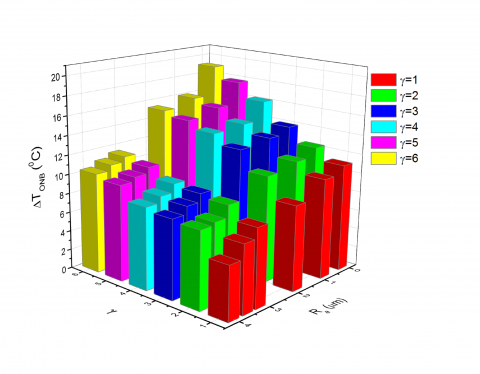Figure 7. Variation in ∆TONB with γ and Ra

The time interval between ONB and CHF i.e. period of boiling regime is estimated from the video recorded during each trial. It is noticed that time interval ∆t between the moment of ONB and CHF decreases with increase in γ, as shown in Figure 8. It is also found that at certain time constant, period of boiling regime increases with increase in Ra. The higher surface roughness resulted in the increase in the mean spacing between two scratches. Hence bubble coalescence retards. Moreover liquid supply significantly increased due to improved surface wettability.

It is noticed in the literature that most of the experiments are carried by supplying unsteady heat supply to thin elements. The boiling characteristics between lumped and non-lumped test sample differs as heat capacity of non-lumped sample has an influence on transient pool boiling. Though the time evolution of the heat supply is identical, the physical mechanism changes with a change in surface roughness. The time interval between the start of heat supply and ONB significantly drops with an increase in Ra. Thus, stored energy in the sample before ONB at constant γ decreases with increase in Ra. It is observed that stored energy and Ra of the sample influences the temperature overshoot (Tov). The sudden drop in wall temperature can be observed for a moderately rough sample as enough number of bubbles can be formed to carry the heat from the sample. For a smooth surface, stored energy before ONB is high enough whereas the number of nucleating bubble are inadequate. Hence Tov is not observed at ONB of a smooth surface.

## 8.png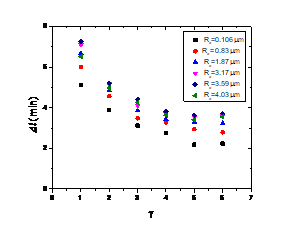Figure 8. Variation in the time interval between ONB and CHF with γ

The variation in the incipience heat flux qONB with γ is plotted for different Ra in Figure 9. It is observed that qONB for smooth and moderately smooth surface increases with increase in γ whereas it is almost constant for highly rough surfaces. Heat flux at ONB from surfaces of high roughness value is found to be approximately 15 % of $q_{C H F, t s}^{\prime \prime}$.

## 9.png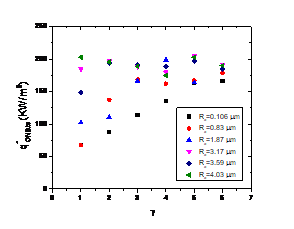Figure 9. Variation in with γ

The transition from the fully developed nucleate boiling (FDNB) regime to the film boiling results in a drop of HTC. It is observed during high-speed visualization that vertical coalescence is followed by the horizontal one which finally turns to the vapor blanket formation on the boiling surface. The CHF of pool boiling is the one which corresponds to the maximum HTC. The HTC drops after the CHF point due to the vapor film. These transient CHF and hmax values for different Ra and γ are plotted in Figure 10 and Figure 11, respectively. It is noticed that transient CHF and hmax are functions of both Ra and γ. Transient CHF and hmax decrease slightly with increase in γ of exponential heat supply whereas it increase remarkably with increase in Ra. The thermo-hydrodynamic instability in the boiling mechanism occurs at rapid exponential heat supply. Vertical coalescence boosts during rapid heat supply due to a drastic drop in the waiting period between two consecutive bubbles and column of vapor forms on the nucleation site. This leads to inadequate liquid supply to nucleation site which leads to liquid dry-out. Thus it is commented that the rate of exponential heating which affects the time of ONB, a period of nucleate boiling and FDNB has influence on the occurrence of CHF. The maximum CHF from all the set of trials is observed for Ra=4.03 μm and γ=1 which is equal to 1532.03 kW/m2. For Ra=4.03 μm, CHF Enhancement during slow transient (γ=1) and rapid transient (γ=6) compared to Ra=0.106 μm is found to be 98.88 % and 91.15 %, respectively. CHF for Ra=0.106 μm, Ra=0.83 μm, Ra=1.87 μm, Ra=3.17 μm, Ra=3.59 μm and Ra=4.03 μm during rapid transient of γ=6 is decreased by 13.43 %, 4.79 %, 9.6 %, 12.76 %, 8.29 % and 16.79 %, respectively, from the CHF obtained during slow transient of γ=1.

## 10.png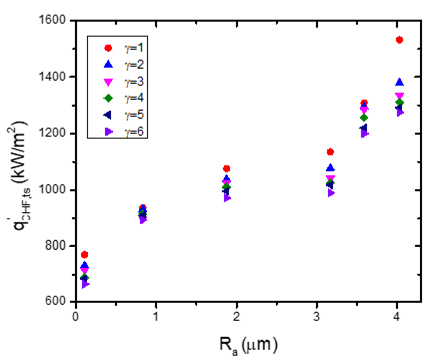Figure 10. Variation in with Ra

## 11.png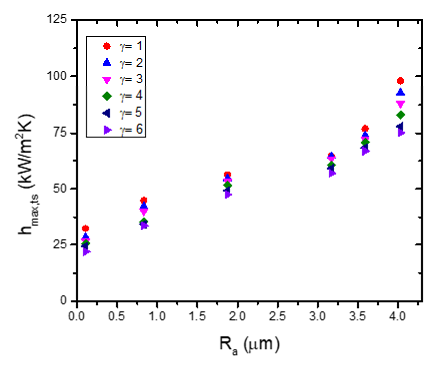Figure 11. Variation in with Ra

The hmax,ts for the samples of Ra=0.106 μm, Ra=0.83 μm, Ra=1.87 μm, Ra=3.17 μm, Ra=3.59 μm and Ra=4.03 μm during rapid transient of γ=6 is decreased by 31.99 %, 24.77 %, 15.42 %, 6.54 %, 12.71 % and 23.26 %, respectively, from hmax,ts obtained during slow transient of γ=1. Non-dimensional h and non-dimensional q are estimated from the reference value of h0 and $q_{0}^{\prime \prime}$ as given in Eq. (5).

$h_{0}=\frac{\mu_{l} c_{p l}}{\operatorname{Pr}_{l}}\left[\frac{g\left(\rho_{l}-\rho_{v}\right)}{\sigma}\right]^{1 / 2}, q_{0}^{\prime \prime}=\mu_{l} h_{f g}\left[\frac{g\left(\rho_{l}-\rho_{v}\right)}{\sigma}\right]^{1 / 2}$ (5)

The variation in the boiling performance with the state of heat supply viz. steady and transient is studied by comparing steady state results with that of transient results of γ=3 for different values of Ra. It is observed in Figure 12 that the heat flux and HTC during the transient heat supply is lower than that of steady-state heat supply for the smooth sample of Ra=0.106 μm. An attempt is made in the present work to enhance the heat flux and HTC by increasing the surface roughness. It is noted that HTC has considerably increased with increase in the heat flux and also with an increase in surface roughness. It is observed that heat flux and HTC in transient state at γ=3 for the sample of Ra=3.17 μm, Ra=3.59 μm, and Ra=4.03 μm are higher than that for the steady state condition for smooth sample of Ra=0.106 μm. It clearly indicates the heat dissipation potential of highly rough surface during the transient heating. It is concluded that the adverse effect of transient heating on the heat flux and HTC can be reduced by increasing surface roughness.

## 12.png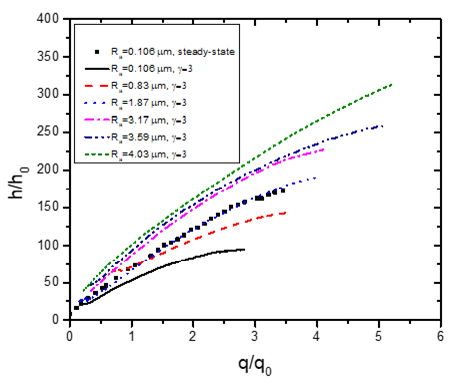Figure 12. Variation in h/h0 with q/q0

4. Development of Correlation

In the present work, correlations are developed based on the heating and boiling surface conditions. The empirical correlation of hmax,ts, as given in Eq. (6), is based on transient CHF, Ra, and γ.

$h_{\max , t s}=0.04(\gamma)^{-0.07}\left(R_{a}\right)^{0.17}\left(q_{C H F, t s}^{\prime \prime}\right)^{1.04}$ (6)

The reduction in CHF due to transient heat supply, defined as $\left(q_{C H F, s s}^{\prime \prime}-q_{C H F, t s}^{\prime \prime}\right) / q_{C H F, s s}^{\prime \prime}$, is plotted against γ in Figure 13 and an empirical correlation is developed as given in Eq. (7). The error between experimental and predicted values obtained from Eq. (7) is found to be ±10 % for different γ, as shown in Figure 14.

$\left(\frac{q_{C H F, s s^{\prime}}^{\prime \prime}-q_{C H F, t s}^{\prime \prime}}{q_{C H F, s s}^{\prime \prime}}\right)=0.19 \times(\gamma)^{0.17}$ (7)

Rohsenow  developed a correlation, given in Eq. (8), to account for the wide variety of surface- fluid combination and pressure over the rate of heat transfer during boiling. It requires empirical factors obtained from the experimental data to account for the surface-fluid combination.

$C_{s f}\left\{\frac{q^{\prime \prime}}{\mu_{l} h_{f g}}\left[\frac{\sigma}{g\left(\rho_{l}-\rho_{v}\right)}\right]^{1 / 2}\right\}^{a} \times \operatorname{Pr}_{l}^{s}=\left[\frac{c_{p l \Delta T}}{h_{f g}}\right]$ (8)

## 13.png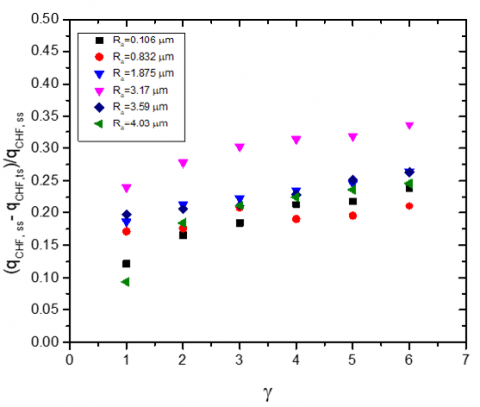Figure 13. Relationship between steady-state and transient CHF

## 14.pngFigure 14. Error between experimental values of CHF reduction and predicted values from Eq. (6)

In Eq. (7), Csf is the surface-fluid combination coefficient and exponents a and s to account for pressure effects. Rohsenow suggested the value of a=0.33 and s=1 for water. The non-dimensional form of the above correlation is given in Eq. (9).

$\frac{h_{t s}}{h_{0}}=\left(\frac{1}{C_{s f}}\right)\left(\frac{q_{t s}^{\prime \prime}}{q_{0}^{\prime \prime}}\right)^{n}$ (9)

where n=1-a

For the present experimental condition, Csf, a surface-fluid coefficient, can be only a function of Ra. Thus the term Csf is given as in Eq. (10) where A is the constant and Ra0=0.4 μm which is suggested by Gorenflo .

$\frac{1}{C_{s f}}=f\left(R_{a}\right)=A\left(\frac{R_{a}}{R_{a_{0}}}\right)^{m}$ (10)

Thus the form of correlation becomes as given in Eq. (11).

$\frac{h_{t s}}{h_{0}}=A\left(\frac{R_{a}}{R_{a_{0}}}\right)^{m}\left(\frac{q_{t s}^{\prime \prime}}{q_{0}^{\prime \prime}}\right)^{n}$ (11)

It is already discussed that hts is a function of surface property i.e. Ra and the exponential heating condition i.e. γ. To account for the present transient condition, constant A in Eq. (11) is modified as a function of γ, as given in Eq. (12).

$A=A^{\prime}(\gamma)^{k}=A^{\prime}\left(\frac{t}{\tau}\right)^{k}$ (12)

Thus the final form of the correlation, as given in Eq. (13), where non-dimensional dependent term

is predicted from the experimental parameters of γ, Ra, and $q_{t s}^{\prime \prime}$.

$\left(\frac{h_{t s}}{h_{0}}\right)=A^{\prime}(\gamma)^{k}\left(\frac{R_{a}}{R_{a_{0}}}\right)^{m}\left(\frac{q_{t s}^{\prime \prime}}{q_{0}^{\prime \prime}}\right)^{n}$ (13)

The experimental values of hts/ho are plotted against each independent term and the exponent is obtained by least square regression. Thus a correlation to predict HTC of distilled water during pool boiling on the rough surface under exponential heat supply is given in Eq. (14).

$\left(\frac{h_{t s}}{h_{0}}\right)=64.612\left(\frac{\tau}{t}\right)^{0.12}\left(\frac{R_{a}}{R_{a_{0}}}\right)^{0.23}\left(\frac{q_{t s}^{\prime \prime}}{q_{0}^{\prime \prime}}\right)^{0.65}$ (14)

The predicted values of hts/ho from the developed correlation are compared with the experimental hts/ho as shown in Figure 15, and it is observed that the correlation predicts the experimental values with a mean absolute error of 9.62 %.

## 15.pngFigure 15. Comparison between predicted and experimental values of non-dimensional hts

5. Bubble Dynamics

High speed camera is synchronized with the programmable power supply to observe the boiling stages and to record the video acquisition rate of 1000 fps with 480×240 pixel resolution. In present study the bubble dynamics of water on unidirectional scratches of wide range of Ra is carried for quasi-steady heat supply i.e. γ =1. The variation in the Jakob number at different heat fluxes at γ =1 for all the test sample is shown in Figure 16. It is observed that Jakob number, (Ja)= , reduced significantly with increase in Ra which justifies the cooling potential of unidirectional scratches. An empirical relation between Jakob number Ja and is given for Ra=0.106 μm and Ra=4.03 μm in Eq. 15.

For $R_{a}=0.106 \mu \mathrm{m} : \quad J a=19 \times q_{t s}^{\prime \prime} 0.2$ (15a)

For $R_{a}=4.03 \mu \mathrm{m} : \qquad J a=1.3 \times q_{t s}^{\prime \prime} 0.5$ (15b)

## 16.png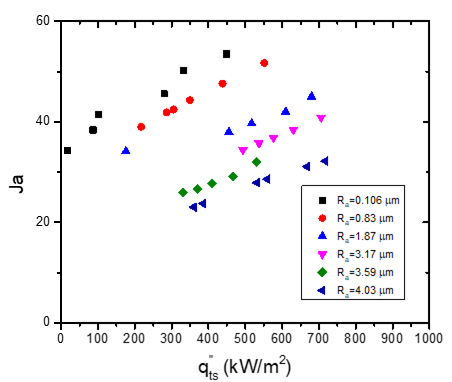Figure 16. Variation of Ja with for all test sample at γ=1

Bubble departure is identified in the recorded videos and the relevant image is selected. Image processing is carried to measure the vertical and horizontal length of the bubble meniscus. The average of that is considered as the equivalent bubble diameter. Thus bubble departure diameter of each sample for different heat flux is given in Figure 17. It is observed that bubble departure diameter significantly increases with increase in surface roughness. As discussed in Walunj and Sathyabhama  the absolute cavity depth (Rz) and average roughness value (Ra) increases with larger grit sandpaper. The radius of bubble nuclei increases with increase in cavity mouth size. Hence it is observed that rough sample can generate larger bubble. Bubble frequency is estimated by considering bubble growth period and waiting time between two consecutive nucleated bubbles from the same site. Compare to smooth surface, unidirectional scratches facilitate the improved liquid supply as each scratch will act as a liquid feeder tube to the growing bubble. This mechanism reduces bubble growth period. Capillary wicking is the ability of the liquid to flow through the narrow passage without external force due to presence of adhesive forces between liquid and solid. It plays vital role during each bubble cycle. The quick liquid replenishment takes place on the departure of bubble. Fraction of vapor entraps in the cavity as liquid exerts capillary force on the bubble neck. This dynamics becomes rapid with increase in surface temperature. Thus waiting time between two consecutive bubbles at the same nucleation site reduced with increase surface roughness. The variation in the bubble frequency with Ja is shown in Figure18. The bubble frequency increased due to reduction in the bubble growth period and bubble waiting period.

## 17.png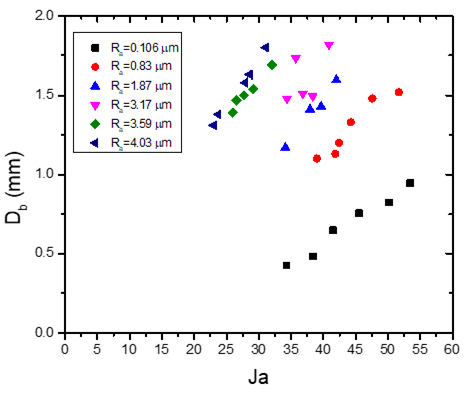Figure 17. Variation of Db with Ja at γ=1

## 18.png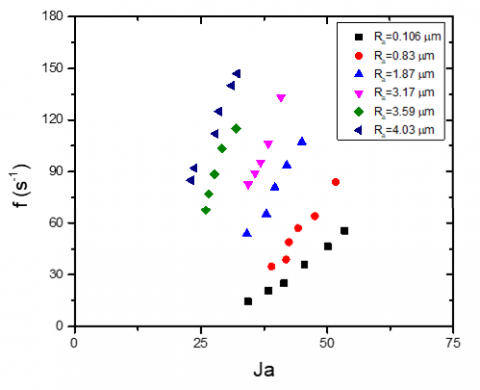Figure 18. Variation of f with Ja at γ=1

6. Conclusion

An extensive study to understand transient pool boiling characteristics of the rough and thick sample under exponentially increasing heat supply at saturated condition is carried. The samples having surface roughness (Ra) ranging from 0.106 μm to 4.03 μm are experimentally tested under variable heat supply condition. Heat supply changes exponentially with different time constant (γ) varied from 1 to 6. The surface roughness effects and exponential transients on ONB temperature, transient CHF, and maximum HTC is examined and following comments are made. A unified correlation is developed for HTC during pool boiling of water accounting the rate of exponential heat supply and surface roughness. Bubble dynamics of water in unidirectional scratches is also studied through high speed visualization.

• It is observed that transient boiling characteristics are highly influenced by the surface structure and rate of exponential heat supply. It is found that CHF during transient heat supply increases with increase in Ra whereas it decreases with increase in γ.
• Enhancement in CHF during slow transient (γ=1) and rapid transient (γ=6) for Ra=4.03 μm is found to be 98.88 % and 91.15 %, respectively.
• Increase in surface roughness resulted in the decrease in ∆TONB however it increases with increase in γ. It is observed that qONB of the smooth and moderately smooth surface increases with increase in γ whereas it is almost constant for highly rough surfaces.
• The developed correlation for HTC predicts the present experimental HTC with MAE of 9.62 %.
• Bubble diameter at the departure and its frequency remarkably increased with increase in the surface roughness.
Nomenclature
 A constant a exponent in the Rohsenow correlation C constant Cp specific heat, J/kg K Csf coefficient in the Rohsenow correlation g gravity, m/s2 h heat transfer coefficient, W/m2K hfg latent heat, J/kg k exponent of time constant kcu thermal conductivity of copper, W/mK m exponent of surface roughness n exponent of heat flux Pr Prandtl number Q heat input,  W q’’ heat flux, W/m2 Ra average roughness, μm Rq root mean squared roughness, μm rc cavity mouth radius, mm T temperature, °C t time, s x distance, mm Greek symbols ∆T wall superheat, °C τ exponential period, s μ viscosity, N s/m2 ρ density, kg/m3 σ surface tension, N/m Subscripts 0 reference CHF critical heat flux l liquid ov overshoot ONB onset of nucleate boiling ss steady state ts transient state v vapor w wall
References

 Rosenthal, M.W. (1957). An experimental study of transient boiling. Nuclear Science and Engineering, 2(5): 640–656. https://doi.org/10.13182/NSE57-A25431

 Johnson, H.A. (1971). Transient boiling heat transfer to water. International Journal of Heat and Mass Transfer, 14(1): 67-82. https://doi.org/10.1016/0017-9310(71)90141-4

 Fukuda, K., Shiotsu, M., Sakurai, A. (1995). Transient pool boiling heat transfer due to increasing heat inputs in subcooled water at high pressures. In: Proceedings of the 7th International Meeting on Nuclear Reactor Thermal Hydraulics, vol. 1. Saratoga Springs, USA, pp. 554–573.

 Sakurai, A., Shiotsu, M., Hata, K., Fukuda, K. (2000). Photographic study of transition from non-boiling and nucleate boiling regime to film boiling due to increasing heat inputs in liquid nitrogen and water. Nuclear Engineering and Design, 200(1-2): 39–54. https://doi.org/10.1016/S0029-5493(99)00325-8

 Fukuda, K., Shiotsu, M., Sakurai, A. (2000). Effect of surface conditions on transient critical heat fluxes for a horizontal cylinder in a pool of water at pressures due to exponentially increasing heat inputs. Nuclear Engineering and Design, 200(1-2): 55–68. https://doi.org/10.1016/S0029-5493(99)00326-X

 Deev, V.I., Oo, H.L., Kharitonov, V.S., Kutsenko, K.V., Lavrukhin, A.A. (2007). Critical heat flux modeling in water pool boiling during power transients. International Journal of Heat and Mass Transfer, 50(19-20): 3780–3787. https://doi.org/10.1016/j.ijheatmasstransfer.2007.02.016

 Duluc, M.C., Stutz, B., Lallemand. M. (2004). Transient nucleate boiling under stepwise heat generation for highly wetting fluids. International Journal of Heat and Mass Transfer, 47(25): 5541–5553.  https://doi.org/10.1016/j.ijheatmasstransfer.2004.04.038

 Su, G.Y., Bucci, M., McKrell, T., Buongiorno, J. (2016). Transient boiling of water under exponentially escalating heat inputs. Part I: Pool boiling. International Journal of Heat and Mass Transfer, 96: 667-684. https://doi.org/10.1016/j.ijheatmasstransfer.2016.01.032

 Balakin, B.V., Delov, M.I., Kuzmenkov, D.M., Kutsenko, K.V., Lavrukhin, A.A., Marchenko, A.S. (2017). Boiling crisis in cryogenic fluids during unsteady heat supply. International Journal of Heat and Mass Transfer, 111: 1107–1111. https://doi.org/10.1016/j.ijheatmasstransfer.2017.04.101

 Htet, M.H., Fukuda, K., Liu, Q.S. (2016). Transient boiling critical heat flux on horizontal vertically oriented ribbon heater with treated surface condition in pool of water. Mechanical Engineering Journal, 3(3): 1-19. https://doi.org/10.1299/mej.15-00438

 Walunj, A., Sathyabhama, A. (2018). Comparative study of pool boiling heat transfer from various microchannel geometries. Applied Thermal Engineering, 128: 672-683. https://doi.org/10.1016/j.applthermaleng.2017.08.157

 Walunj, A., Sathyabhama, A. (2018). Transient CHF enhancement in high pressure pool boiling on rough surface. Chemical Engineering and Processing – Process Intensification, 127: 145-158. https://doi.org/10.1016/j.cep.2018.03.025

 Griffith, P., Wallis, J. (1960). The role of surface condition in nucleate boiling. Chem. Eng. Prog. Symp. Ser., 56: 49–63.

 Rohsenow, W.M. (1952). A method of correlating heat transfer data for surface boiling of liquids. Trans. ASME, 74: 969–976. http://hdl.handle.net/1721.1/61431

 Gorenflo, D. (1993). Pool Boiling, VDI Heat Atlas, VDI Verlag, Düsseldorf.

 Walunj, A., Sathyabhama, A. (2018). Bubble dynamics and enhanced heat transfer during high-pressure pool boiling on rough surface. Journal of Thermophysics and Heat Transfer, 33(2): 1-13. https://doi.org/10.2514/1.T5495